# Binary Symmetric Channel

Introduce binary errors

•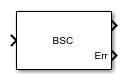Libraries:
Communications Toolbox / Channels

## Description

The Binary Symmetric Channel block introduces errors to the input signal transmitted through a binary symmetric channel. The errors are introduced based on the specified Error probability. For more information, see Tips.

## Examples

expand all

The `cm_ex_viterbi_decode_binary_seq` model generates a binary sequence using the `Random Integer Generator` block. The sequence is encoded with the `Convolutional Encoder` block and then impaired with the `Binary Symmetric Channel` block. The `Viterbi Decoder` block decodes the data sequence and the bit error rate is computed.

The `InitFcn` callback is used to initialize workspace parameters for samples per frame, BSC error probability, and the Viterbi decoder traceback depth. The signal delay between the transmitted and received signal is equal to the traceback depth. The signal delay is needed for the error rate calculation.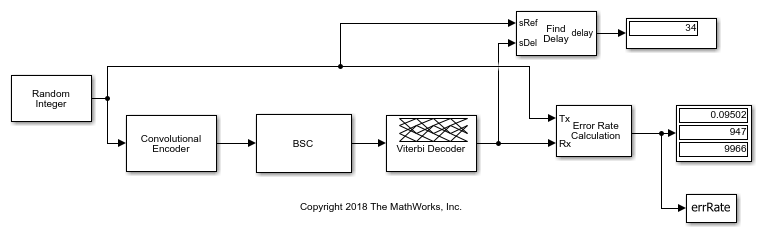To produce a binary bit stream, the `Random Integer Generator` block specifies a set size of `2`, and output type of `boolean`.

The computed error rate approximates the `Error probability` specified in the `Binary Symmetric Channel` block.

```Computed error rate = 0.095023 ```

Pass a random signal through a binary symmetric channel. Calculate the error rate for specified error probability.

Use the Open model button to open the `slex_bsc_channel` model.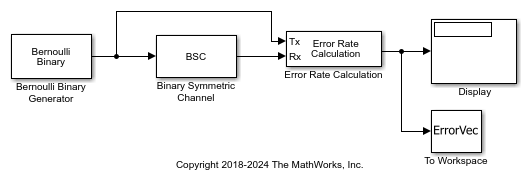Run the model with the error probability of the BSC block set to 0.01. The results are saved to the base workspace variable `ErrorVec` in a 1-by-3 row vector. The first element contains the BER.

```With error probability set to 0.01, BER: 0.009929 ```

Change the error probability of the BSC block to 0.04. Run the model and observe the increase in BER.

```With error probability set to 0.04, BER: 0.039889 ```

Pass a random signal through a binary symmetric channel. For a specified error probability, compare the total number of errors indicated by the `Err` port to result reported by the `Error Rate Calculation` block.

Open the `slex_bsc_channel_err` model.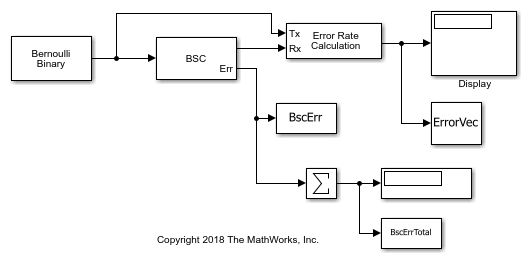Run the model with the error probability of the BSC block set to 0.02. The results from the `Error Rate Calculation` block are saved to the base workspace variable `ErrorVec` in a 1-by-3 row vector. `ErrorVec` contains [ `BER`, `TotalErrors`, `TotalSamplesProcessed` ].

```Total number of errors: 20296 ```

The `Err` port for the `Binary Symmetric Channel` is a binary vector whose length matches the total number of samples processed. By summing the elements of the output `Err` vector you get the total number of errors introduced into the signal data. The sum of the elements of the `Err` vector is saved to the base workspace variable `BscErrTotal`.

```BscErrTotal: 20296 ```

The `Error Rate Calculation` blocks reports the same total number of errors indicated by `BscErrTotal`.

Pass a multi-channel random signal through a binary symmetric channel. For a specified error probability, compare the error rate comparing the `Err` port output for each channel.

Use the Open model button to open the `slex_multichannel_bcs_err` model.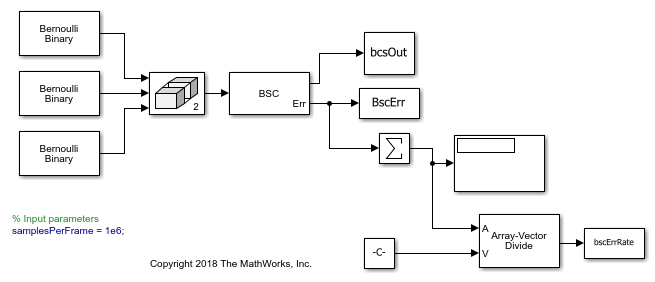Set different BSC error probabilities for the individual channels of a multi-channel signal by defining the `Error probability` as a row vector set to [ `0.02 0.04 0.07` ].

The `Err` port for the `Binary Symmetric Channel` is a binary matrix whose dimensions match the input signal. `Err` contains the error results for each channel. Sum the elements of each column output in `Err` individually to get the total number of errors introduced per channel. Dividing each total by the total number of samples sent per channel yields the bit error rate for each channel.

```BER for channel 1: 2.02960e-02 BER for channel 1: 3.97430e-02 BER for channel 1: 7.00090e-02 ```

The computed BERs are approximately equal to the probabilities set in the `Error probability` parameter.

## Ports

### Input

expand all

Input signal, specified as a column vector or an NS-by-NC matrix of `Boolean` values. NS is the number of samples per channel. NC is the number of independent data channels. For more information, see Tips.

### Output

expand all

Binary output signal, returned as a column vector or matrix with the same dimensions as `Input`. The output signal is a version of the input signal that has been modified by introducing random errors based on the specified Error probability. To set the output data type, use Output data type.

Error locations, returned as a column vector or matrix with the same dimensions as `Input`. Element values in `Err` are `1` or `0`, where:

• `1` indicates that the corresponding element in `Output` has an error.

• `0` indicates that the corresponding element in `Output` does not have an error.

The data type of `Err` is the same as Output, as set by Output data type.

#### Dependencies

To enable this port, select Output error vector.

## Parameters

expand all

To edit block parameters interactively, use the Property Inspector. From the Simulink® Toolstrip, on the Simulation tab, in the Prepare gallery, select .

Probability of error occurrence for the input signal elements, specified as a scalar in the range [0,1]. The probability of error applies independently for each element.

To enable the `Err` output port to the block, select this parameter.

Select the output data type as `double`, `single`, or `boolean`. This parameter sets the output data type for both the Output and Err ports.

Initial seed value for the random number generator used by the block, specified as an integer. The block uses the `mt19937ar` algorithm to generate uniformly distributed random numbers. For details about the `mt19937ar` algorithm, see Creating and Controlling a Random Number Stream.

Type of simulation to run, specified as:

• `Code generation` –– Simulate the model using generated C code. The first time you run a simulation, Simulink generates C code for the block. The C code is reused for subsequent simulations as long as the model does not change. This option requires additional startup time.

• `Interpreted execution` –– Simulate the model using the MATLAB® interpreter. This option shortens startup time. In `Interpreted execution` mode, you can debug the source code of the block.

## Block Characteristics

 Data Types `Boolean` | `double` | `fixed point` | `integer` | `single` Multidimensional Signals `no` Variable-Size Signals `no`

## Tips

• When the input consists of not `Boolean` values, Binary Symmetric Channel converts zero-valued elements to 0 and converts nonzero-valued elements to 1.

• The Binary Symmetric Channel block creates and uses an independent `RandStream` to provide a random number stream for probability determination.

• To generate repeatable results, use the same Initial seed value.

• To generate independent probability statistics, set different Initial seed values for multichannel signals, multiple processing chains, or simulation runs.

## Version History

Introduced before R2006a

expand all